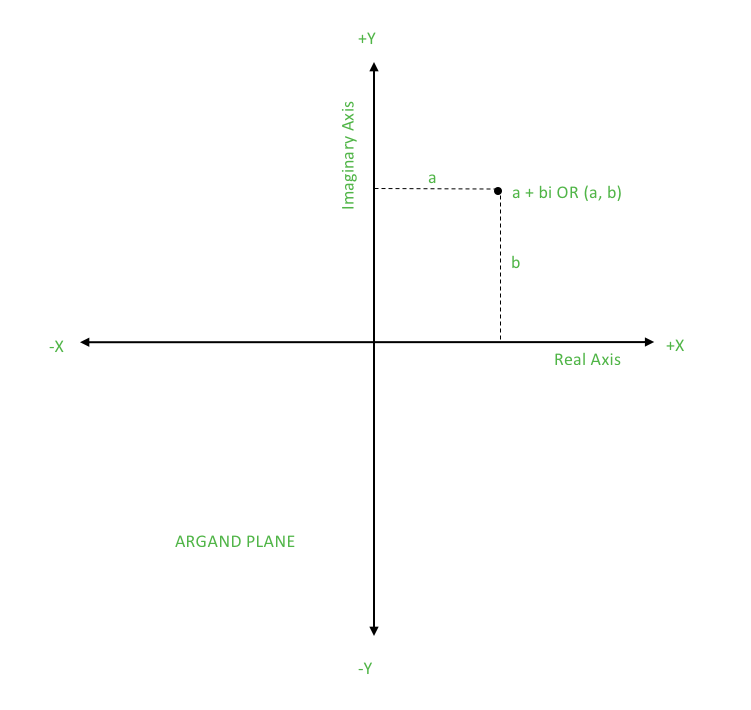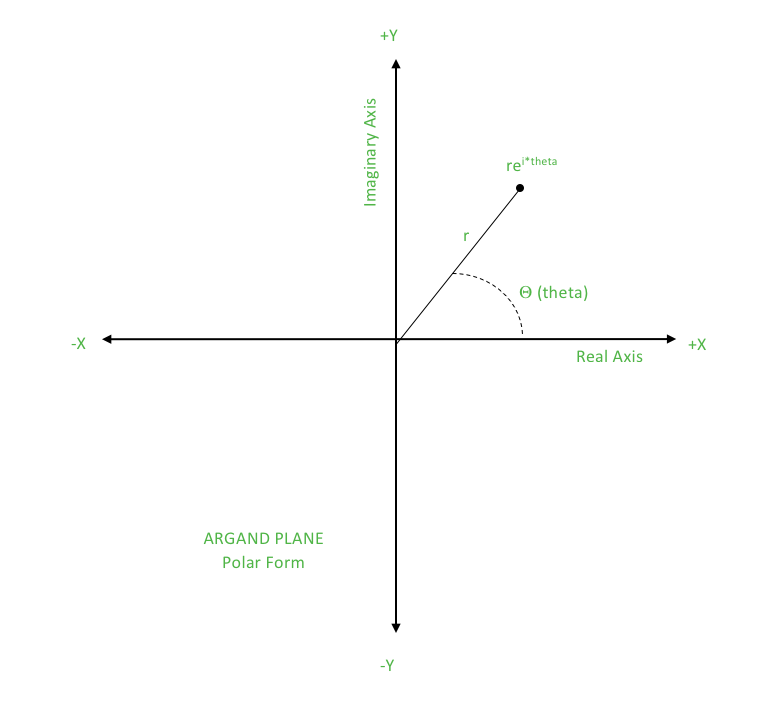Open In App

# Geometry using Complex Numbers <std::complex> in C++ | Set 1

While solving geometric problems, it is time consuming to define the point class for specifying a point on the 2D Plane or the Euclidean Plane. So, this article specifies a faster and clever way to implement the same using complex class from STL in C++.
Before implementation, it is essential to understand what are complex numbers and how they can help in representing a point on a 2D Plane.

What is Complex Number?

Complex numbers are of the form

```a + bi
where, a is the real part
b is the imaginary part```As we can see from the diagram, a complex number can be represented on the 2D Plane. Thus for a point (a, b), we can have a complex number a + bi where a is the X-coordinate and b is the Y-coordinate.
Important Note: i2 = -1

• Polar Form of Complex Number: An alternative way of visualizing and representing complex numbers is the polar form. The polar form uses the magnitude of the complex number in form of ‘r’ and the direction of complex number in form of ‘θ’.
A complex number with these parameters is then r(cosθ + isinθ).
Note: θ is considered to be in radians.

re = r(cosθ + isinθ)• Conjugate of a Complex Number:
If z = a + bi, then the conjugate of z is z’ = a – bi.
If z = (r, θ), then the conjugate of z is z’ = (r, -θ)
Conjugate of a complex number can be used to attain certain special properties as under:

• z + z’ = (a + bi) + (a – bi) = 2a
Real part = (complex number + conjugate)/2
• z – z’ = (a + bi) – (a – bi) = 2b
Imaginary part = (complex number – conjugate)/2
• z*z’ = (a + bi) * (a – bi) = a2 – b2i2 + 2abi – 2abi = a2 + b2
Magnitude2 = complex number * conjugate

How to use Complex numbers?

Let us consider point P (a, b) on the Euclidean Plane. Now we make a complex number z = a + bi and bring out the equivalence between the two. Some attributes associated with P are:

1. The X-Coordinate of P: We can simply say X coordinate = a. Thus, return the real part of z.
2. The Y-Coordinate of P: We can simply say Y coordinate = b. Thus, return the imaginary part of z.
3. The distance of P from origin (0, 0): Distance of P from origin = sqrt((a-0)2 + (b-0)2) = sqrt(a2 + b2)
Magnitude of z = sqrt(a2 + b2)
Thus, return the magnitude of the z.
4. The angle made by OP from the X-Axis where O is the origin: Angle made by OP from the X-Axis = tan-1(b/a)
Argument i.e. the parameter theta of z can be derived as under:
rcosθ = a ….. (i)
rsinθ = b ….. (ii)
Dividing (ii) by (i)
tanθ = (b/a)
θ = tan-1(b/a)
Thus, return the argument of z.
5. Rotation of P about origin: Rotation of a point about origin will not change its distance from the origin but will only change the angle that PO makes with the X-Axis.
So, rotation equivalence can be better understood if we consider the complex number in polar form.
z = re
Let the point be rotated by ‘α’ in the anticlockwise direction.
The point now becomes rei(θ + α) = re * 1e = z * 1e
Thus, return z * polar(1, α).
where, polar(r, θ) is the general representation.

Let us consider points P (a, b) and Q (c, d) on the Euclidean Plane. These can be basically considered to be vectors with the length equal to distance from origin and direction as made with the X-Axis. (Many attributes can be better understood if the points are taken as vectors which is one of the key idea in various geometric algorithms).
Now let us consider that z1 = a + bi and z2 = c + di.
Some attributes associated with P and Q are:

```(a, b) + (c, d) = (a + c, b + d)
z1 + z2 = (a + bi) + (c + di) = (a + c) + (b + d)i```

Thus, return z1 + z2.

2. Vector Subtraction: Simply return
`z1 – z2`
3. Slope of line PQ: The argument of
`z2 – z1 gives the angle of elevation`

So, the slope of the line PQ is given by the tangent of the angle of elevation.
Thus, return tangent of argument of z2 – z1.

4. Euclidean Distance:
```Distance of P from Q = sqrt((a-c)2 + (b-d)2)
Magnitude of z1 – z2 = sqrt((a-c)2 + (b-d)2)```

Thus, return the magnitude of the z1 – z2.

```An important construction which will be used frequently in geometric problems is z1’z2.
Let’s compute this:
z1’ = a – bi
z1’z2 = (a – bi)*(c + di) = ac + adi – bci + bd = (ac + bd) + (ad – bc)i
```
5. Dot Product: Dot product for vectors P and Q is
`(ac + bd)`

This is same as the real part of the above construction. Thus, return the real part of z1’z2.

6. Cross Product: The magnitude of cross product for vectors P and Q is
`(ad – bc)`

This is same as the imaginary part of the above construction. Thus, return the imaginary part of z1’z2.

The implementation part for the complex class is covered in Set 2

This article is contributed by Aanya Jindal. If you like GeeksforGeeks and would like to contribute, you can also write an article using contribute.geeksforgeeks.org or mail your article to contribute@geeksforgeeks.org. See your article appearing on the GeeksforGeeks main page and help other Geeks.